Latest Banking jobs   »   Quantitative Aptitude Quiz for IBPS 2020...

Quantitative Aptitude Quiz for IBPS 2020 Mains Exams- 31st December

Directions (1-5): There are five shop owners A, B, C, D and E. They are selling four different items given in the table.
In the table, Discount (as a percentage) is given on mark price of these four products by different sellers. Study the table and answer the following questions: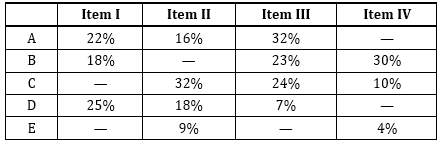Note:
1. Some values are missing. You have to calculate these values as per data given in the questions.
2. Mark price of a particular item is same for all of the shop owners.

Q1. If the profit percentage of seller A after selling item II is s% and that of seller C for the same item is (3s – 2) % and the ratio of cost price of item II by seller A and seller C is 21 : 17 then find the value of s ?
(a) 2
(b) 3
(c) 4
(d) 1
(e) none of these

Q2. For seller D, difference between the selling price of item II and that of item III is 4810 Rs. if the sum of the mark price of item II and item III by the same seller is 8000 then find the Mark price (in Rs.) of item II is how much more (in Rs.) than that of item III for the same seller? (Selling price of item II is greater than that of item III)
(a) 5000
(b) 6000
(c) 6500
(d) 5500
(e) 4500

Q3. Average SP of item II by seller A and B is Rs 4400. Average SP of item II by seller B and C is Rs 3600. Find the SP (in Rs.) of item II by seller C.
(a) 4500
(b) 3600
(c) 5400
(d) 4000
(e) 6800

Q4. If the cost price of item I and selling price of item III by seller E are in the ratio of 5 : 6. If the seller earned a profit of 25% which is Rs. 750 on item I and 20% on item III then find the total profit (in Rs.) by selling item I and item III together by the same seller?
(a) 1250
(b) 2000
(c) 1750
(d) 1350
(e) 1500

Q5. If Cost price of item III is 5400 Rs. for all of the sellers and all of them marked the same product at 100% higher than the cost price, then to get a total profit of 13500 Rs. by all of the five sellers after selling item III, what is the minimum discount (as a percentage) should be provided by seller E on item III.
(a) 15%
(b) 13%
(c) 11%
(d) 20%
(e) None of these

Directions (6-10): The following questions are accompanied by two statements (I) and (II). You have to determine which statements(s) is/are sufficient/necessary to answer the questions.

Q6. Let t be total number of balls in a bag. Balls are of 3 colors – black, white and red. Find t.
(I) when one ball is drawn then Probability of getting a black ball is 1/6, a red ball is 1/6 & a white ball is 2/3.
(II) If one white ball is lost and a ball is drawn, then probability of not getting a white ball is 8/23.

(a) Statement (I) alone is sufficient to answer the question but statement (II) alone is not sufficient to answer the question.
(b) Statement (II) alone is sufficient to answer the question but statement (I) alone is not sufficient to answer the question.
(c) Both the statements taken together are necessary to answer the question, but neither of the statements alone is sufficient to answer the question.
(d) Either statement (I) or statement (II) by itself is sufficient to answer the question.
(e) Statements (I) and (II) taken together are not sufficient to answer the question.

Q7. Shivam and Deepak invested in a partnership business in the ratio of 4 : 5. Find the profit share of Shivam.
(I) Shivam invested Rs.12000 and period of investment of Shivam and Deepak is 10 months and 4 months respectively.
(II) Ratio of period of investment of Shivam and Deepak is 5 : 2 and Deepak’s profit share is Rs.12000 less than Shivam’s profit share.

(a) Statement (I) alone is sufficient to answer the question but statement (II) alone is not sufficient to answer the question.
(b) Statement (II) alone is sufficient to answer the question but statement (I) alone is not sufficient to answer the question.
(c) Both the statements taken together are necessary to answer the question, but neither of the statements alone is sufficient to answer the question.
(d) Either statement (I) or statement (II) by itself is sufficient to answer the question.
(e) Statements (I) and (II) taken together are not sufficient to answer the question.

Q8. Calculate the marked price of item?
(I) Shopkeeper marked the article 80% above its cost price and shopkeeper earned Rs.100 profit on the article.
(II) Ratio of marked price and discount allowed on the article is 3 : 1.

(a) Statement (I) alone is sufficient to answer the question but statement (II) alone is not sufficient to answer the question.
(b) Statement (II) alone is sufficient to answer the question but statement (I) alone is not sufficient to answer the question.
(c) Both the statements taken together are necessary to answer the question, but neither of the statements alone is sufficient to answer the question.
(d) Either statement (I) or statement (II) by itself is sufficient to answer the question.
(e) Statements (I) and (II) taken together are not sufficient to answer the question.

Q9. Calculate the rate of interest.
(I) Pankaj earned Rs.4500 as interest, when he invested Rs.6000.
(II) Pankaj invested equal amount at SI and at CI. After 2 years, CI received by Pankaj is Rs.90 more than the SI received by Pankaj.

(a) Statement (I) alone is sufficient to answer the question but statement (II) alone is not sufficient to answer the question.
(b) Statement (II) alone is sufficient to answer the question but statement (I) alone is not sufficient to answer the question.
(c) Both the statements taken together are necessary to answer the question, but neither of the statements alone is sufficient to answer the question.
(d) Either statement (I) or statement (II) by itself is sufficient to answer the question.
(e) Statements (I) and (II) taken together are not sufficient to answer the question.

Q10. Find the volume of cylinder.
(I) Curved surface area of cylinder is 1760 cm2 and total surface area of cylinder is 70% more than its curved surface area.
(II) Volume of cylinder is twice of that of cone. Radius of cylinder and cone is equal and ratio of height of cylinder to that of cone is 2 : 3. Height of cone is 30 cm.

(a) Statement (I) alone is sufficient to answer the question but statement (II) alone is not sufficient to answer the question.
(b) Statement (II) alone is sufficient to answer the question but statement (I) alone is not sufficient to answer the question.
(c) Both the statements taken together are necessary to answer the question, but neither of the statements alone is sufficient to answer the question.
(d) Either statement (I) or statement (II) by itself is sufficient to answer the question.
(e) Statements (I) and (II) taken together are not sufficient to answer the question.

Directions (11-15): Study the passage given below and answer the following questions.

There are 3 cities – Delhi, Dhaka and Bangkok. Total distance between Delhi – Dhaka, Dhaka – Bangkok and Delhi – Bangkok is 19800 km. Distance between Dhaka – Bangkok is 80% of the distance between Delhi – Bangkok and ratio of distance between Delhi – Dhaka to distance between Bangkok – Dhaka is 27 : 32.
Fares of 3 different flight operators (A, B & C) on these 3 routes (Delhi – Dhaka, Dhaka – Bangkok and Delhi – Bangkok) are –
Delhi – Dhaka: Fare of C is 25% more than that of B and fare of A is Rs.2700 less than that of B. Ratio of fare of A to that of C is 3 : 5.
Delhi – Bangkok: Average fare of A & B is Rs.20000 and fare of C is Rs.8000 more than average fare of A & B. Average fare of B & C is Rs.26000.
Dhaka – Bangkok: Fare of B is Rs.16000 and ratio of fare of A to that of B to that of C is 6 : 5 : 8.

Q11. If Veer wants to travel from Delhi – Dhaka and then Dhaka – Bangkok without changing flight operator, then which flight operator would be the cheapest option among A, B & C for Veer.
(a) A
(b) B
(c) C
(d) Either A or B
(e) Either A or C

Q12. If Deepak is travelling from Dhaka – Bangkok and Bangkok – Delhi from C, then find the amount paid by Deepak per km for the entire trip.
(a) Rs. 3.11/km
(b) Rs. 3.98/km
(c) Rs. 3.42/km
(d) Rs. 3.62/km
(e) Rs. 3.01/km

Q13. For which of the following trip, the fare will be maximum?
(a) A, Delhi – Bangkok
(b) C, Delhi – Dhaka
(c) B, Dhaka – Bangkok
(d) A, Dhaka – Bangkok
(e) B, Delhi – Dhaka

Q14. On Dhaka – Bangkok route, which flight operator is charging lowest fare per km?
(a) A
(b) B
(c) C
(d) Either B or C
(e) Cannot be determined.

Q15. Find average fare of A, B & C on Delhi – Dhaka route.
(a) Rs.12000
(b) Rs.11500
(c) Rs.10400
(d) Rs.11200
(e) Rs.10800

Practice More Questions of Quantitative Aptitude for Competitive Exams:

Mains Quiz Study Plan for IBPS Exams 2020

Solutions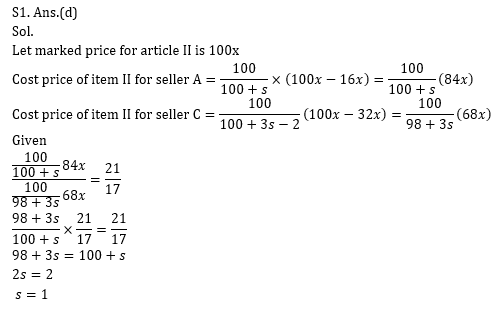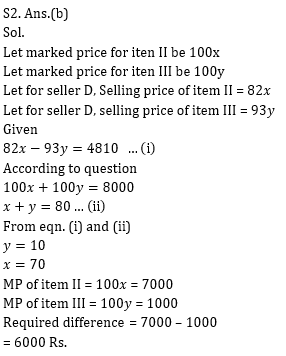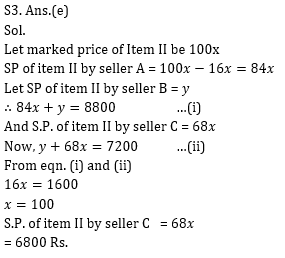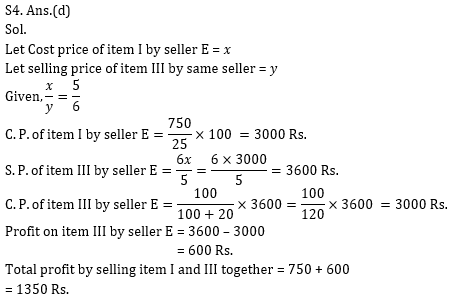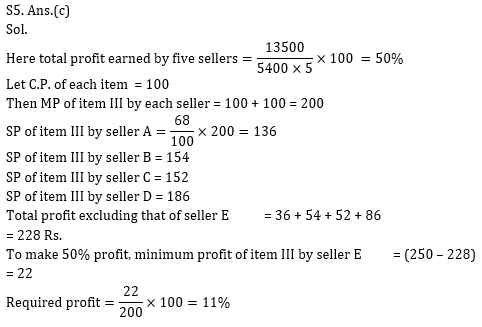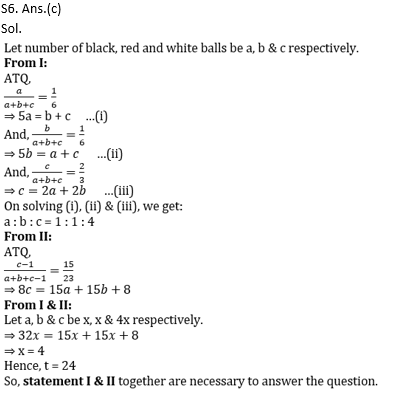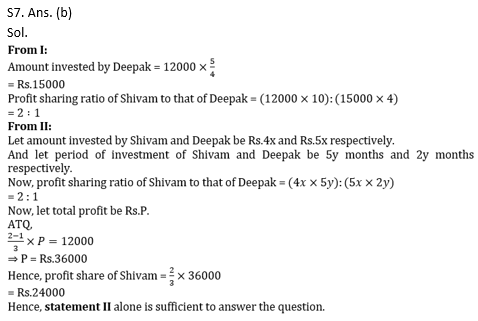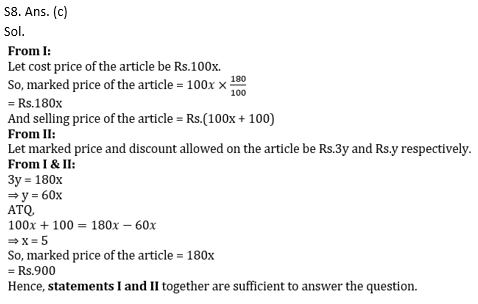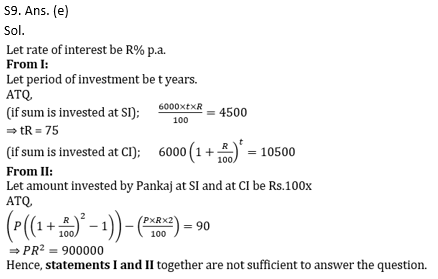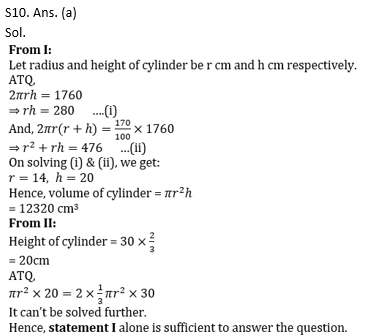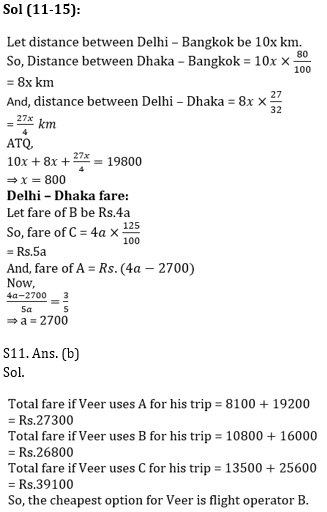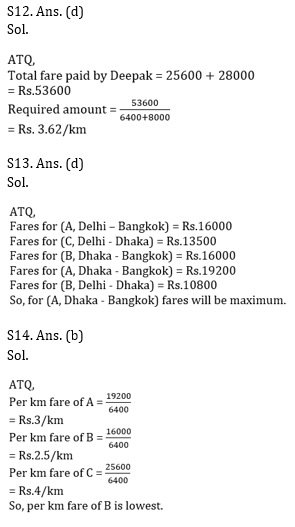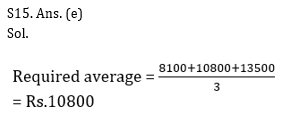Practice with Crash Course and Online Test Series for IBPS Clerk Prelims:×

Thank You, Your details have been submitted we will get back to you.Join India's largest learning destination

What You Will get ?

•Job Alerts
•Daily Quizzes
•Subject-Wise Quizzes
•Current Affairs
•Previous year question papers
•Doubt Solving session

ORJoin India's largest learning destination

What You Will get ?

•Job Alerts
•Daily Quizzes
•Subject-Wise Quizzes
•Current Affairs
•Previous year question papers
•Doubt Solving session

ORJoin India's largest learning destination

What You Will get ?

•Job Alerts
•Daily Quizzes
•Subject-Wise Quizzes
•Current Affairs
•Previous year question papers
•Doubt Solving session

Enter the email address associated with your account, and we'll email you an OTP to verify it's you.Join India's largest learning destination

What You Will get ?

•Job Alerts
•Daily Quizzes
•Subject-Wise Quizzes
•Current Affairs
•Previous year question papers
•Doubt Solving session

Enter OTP

Please enter the OTP sent to
/6

Did not recive OTP?

Resend in 60sJoin India's largest learning destination

What You Will get ?

•Job Alerts
•Daily Quizzes
•Subject-Wise Quizzes
•Current Affairs
•Previous year question papers
•Doubt Solving sessionJoin India's largest learning destination

What You Will get ?

•Job Alerts
•Daily Quizzes
•Subject-Wise Quizzes
•Current Affairs
•Previous year question papers
•Doubt Solving session

Almost there

+91Join India's largest learning destination

What You Will get ?

•Job Alerts
•Daily Quizzes
•Subject-Wise Quizzes
•Current Affairs
•Previous year question papers
•Doubt Solving session

Enter OTP

Please enter the OTP sent to Edit Number

Did not recive OTP?

Resend 60

By skipping this step you will not recieve any free content avalaible on adda247, also you will miss onto notification and job alerts

Are you sure you want to skip this step?

By skipping this step you will not recieve any free content avalaible on adda247, also you will miss onto notification and job alerts

Are you sure you want to skip this step?